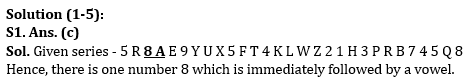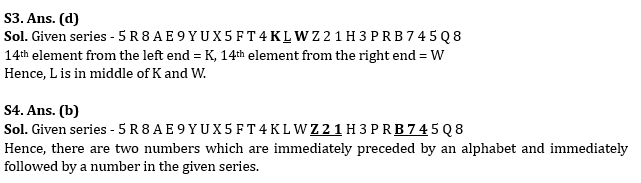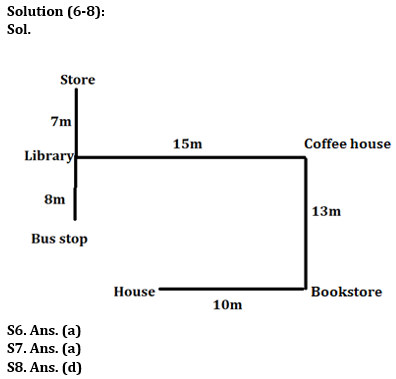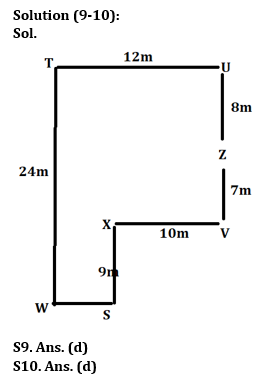Latest Banking jobs   »

# Reasoning Ability Quiz For Bank Foundation 2023 -23rd May

Directions (1-5): Study the following series carefully and answer the questions:

5 R 8 A E 9 Y U X 5 F T 4 K L W Z 2 1 H 3 P R B 7 4 5 Q 8

Q1. In the given series, how many numbers are immediately followed by a vowel?
(a) Two
(b) Three
(c) One
(d) Four
(e) None of these

Q2. In the given series, which of the following element is 8th to the right of 6th number from the left end?
(a) X
(b) 4
(c) B
(d) 3
(e) None of these

Q3. Which element is exactly in the middle of 14th element from left end and 14th element from right end?
(a) X
(b) Z
(c) W
(d) L
(e) None of these

Q4. How many numbers are immediately preceded by an alphabet and immediately followed by a number in the given series?
(a) One
(b) Two
(c) Three
(d) Four
(e) None of these

Q5. Which of the following element is 9th to the left of 15th element from the right end in the given series?
(a) 9
(b) E
(c) Y
(d) 8
(e) None of these

Directions (6-8): Answer the questions based on the information given below.
Amayra leaves her house and moves in east direction. After moving 10m she stops at a bookstore. From there, she moves 13m to her left and stops at a coffee house. From there, she moves 15m to her left and stops at a library. At last, she moves 7m to her right and stops at a store. The library is 8m north of a bus stop.

Q6. What is the shortest distance between Bus stop and Coffee house?
(a) 17m
(b) 18m
(c) 19m
(d) 20m
(e) None of the above

Q7. What is the direction of Store with respect to Bookstore?
(a) North-west
(b) North-east
(c) South-west
(d) South east
(e) None of the above

Q8. If bus stop would be in west direction of bookstore, then what will be the distance between store and bus stop?
(a) 17m
(b) 18m
(c) 19m
(d) 20m
(e) None of the above

Directions (9-10): Answer the questions based on the information given below.
Eight friends S, T, U, V, W, X, Y and Z are sitting in a park at certain distance from each other. T is 12m west of U. Z is 8m south of U and 7m north of V. X is 10m west of V. S is 9m south of X. W is in the west of S and south of T.

Q9. What is the distance between T & W?
(a) 21m
(b) 22m
(c) 23m
(d) 24m
(e) None of the above

Q10. What is the direction of S with respect to T?
(a) North-west
(b) North-east
(c) South west
(d) South east
(e) None of the above

SolutionsS2. Ans. (b)
Sol. Given series – 5 R 8 A E 9 Y U X 5 F T 4 K L W Z 2 1 H 3 P R B 7 4 5 Q 8
6th number from the left end = 2, 8th to the right of 2 = 4S5. Ans. (a)
Sol. Given series – 5 R 8 A E 9 Y U X 5 F T 4 K L W Z 2 1 H 3 P R B 7 4 5 Q 8
15th element from the right end = L, 9th element to the left of L = 9## FAQs

### What is the selection process of the Bank Clerk?

The selection process of the Bank Clerk is Prelims & Mains.

#### Congratulations!Union Budget 2023-24: Free PDF